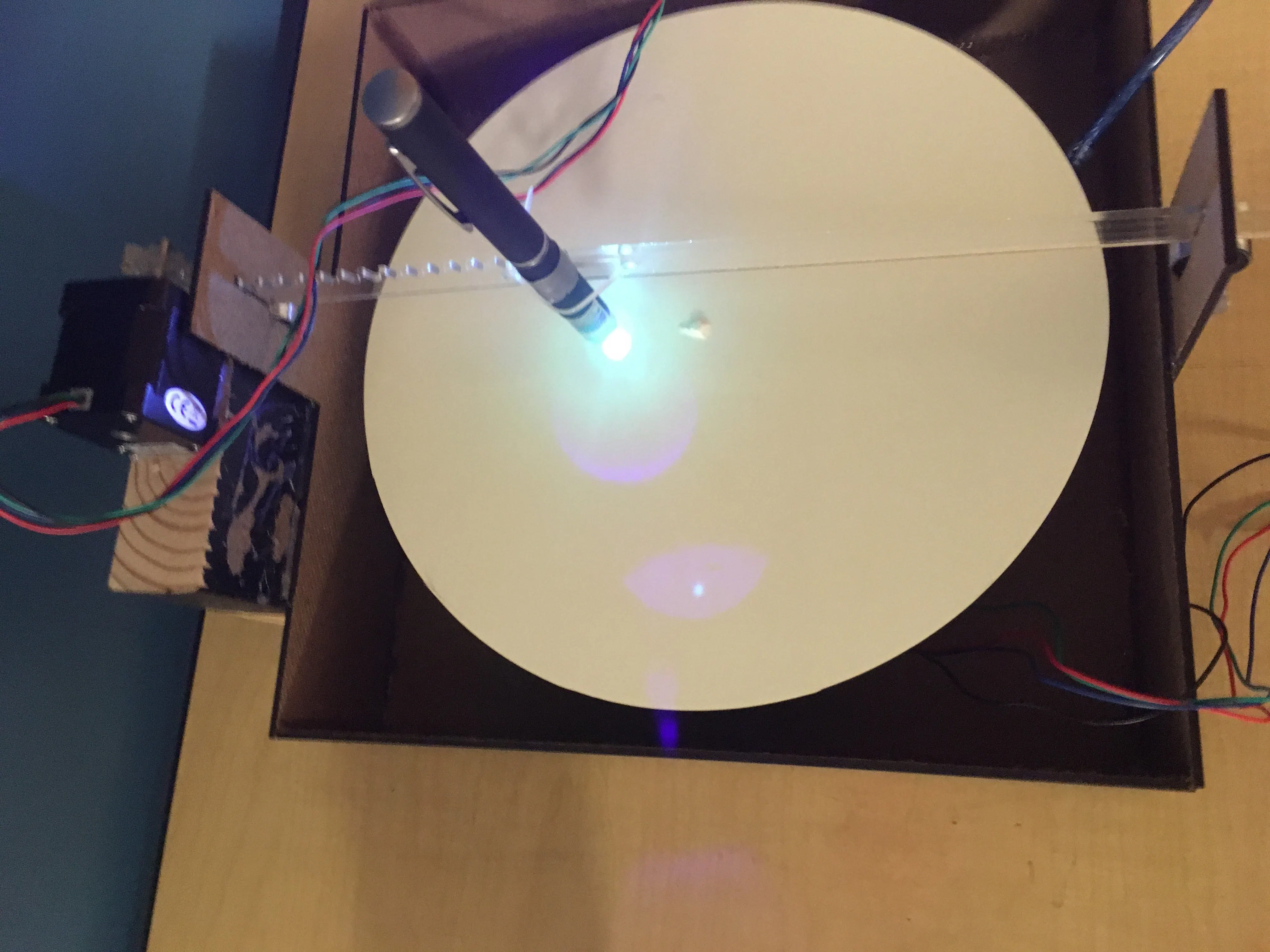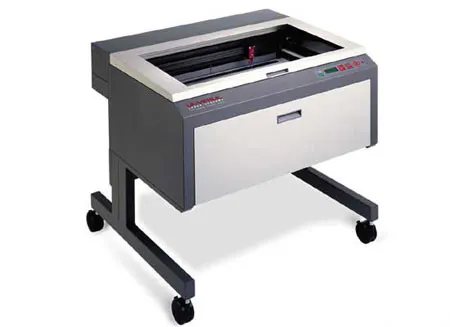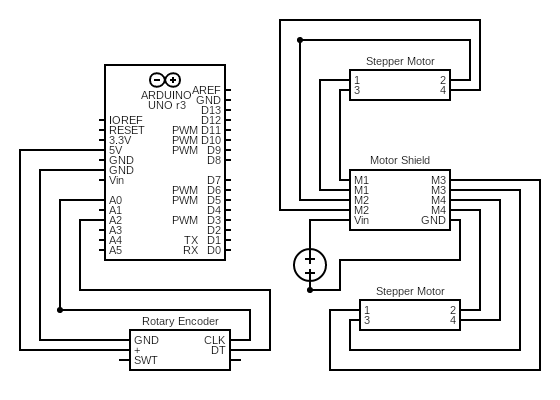Project tutorial# Fourier Box

Using a blue laser and phosphorescent paper to illustrate what it means to sketch a sine wave around a circle.

• 4,981 views
• 7 respects

## Necessary tools and machinesLaser cutter (generic)

## Apps and online services

### The Background:

Have you ever wondered about how sound editing software can cut or boost the base in a recording? Or how sound-canceling headphones work? Or how civil engineers can design buildings and avoid the resonant frequencies of earthquakes? Well, the answer to all of those questions originates with one man: Joseph Fourier. Fourier figured out how to mathematically brake a complex wave down into its component sine waves, allowing each to be edited or observed individually. The general idea (without getting into the calculus behind it) is that you take the wave and wrap it around a circle so that it draws a type of flower. You then gradually increase the frequency at which you wind the wave around that circle, watching the shapes that the graph draws change. You do this until you find a frequency that draws something that looks like a heart shape. When you find that, it means that the frequency at which you are winding the wave around the circle is the same as the frequency of one of the component sine waves in the parent wave you are analyzing. I decided to make a physical representation of that process of finding the component sine waves.

### The Project:

The idea that we (Henry Haggart and I) came up with is to have a rotating circle of phosphorescent paper which you can control the speed of under a linear actuator with a blue laser attached to it. The idea is that the linear actuator with the laser would trace a sine wave as you altered the speed at which the circle turned to try and find where they matched and you started to draw hearts instead of flowers. This could be easily achieved by driving two separate stepper motors and using the input from a rotary encoder to change the speed at which the base circle rotates. The rest of the physical design was relatively rudimentary, as we only needed a way to suspend the laser above the base circle on a custom fabricated linear actuator which we laser cut from acrylic.

### Explaining the Code:

Programming this device, while it wasn’t excessively hard, did present some unexpected challenges for me. Firstly, I had never worked with Arduino and steppers, and so did not think about the mechanics of the setSpeed function. What the speed does is just add a different delay between steps in the program. This was a problem for us, as every time the delay changed in the sine wave generator code, it would also change the speed of the base circle. As it was getting dangerously close to exhibition time, the quick fix was to simply run the two motors off of two different boards. This was a way to make the code much more readable and (more importantly) debuggable. Later on, however, I learned about a way to run the two codes together on the same Arduino without having the delay in the sine function effect the delay in the base circle.

Here is the code snippet that allowed us to do that:

``````if ((millis() - previousMillis) >= value){
motor2.step(1, FORWARD, DOUBLE);
previousMillis = millis();
}
``````

The millis() function in the Arduino IDE is a timer function, so its value counts at a constant rate no matter the delays that you may have in your code. What this does is looks for when millis() has counted up a certain increment (value or your base circle speed) and when that condition is met, it takes one step forward with the base stepper motor.

## Code

##### Arduino 1 : Sine WaveArduino
```double ti;
double inp;
double cosine;
double nn;
double pi = 3.1415926535;
int sinSpeed = 126;  // How fast the arm is moving back and forth

#include <AFMotor.h>

AF_Stepper motor(200, 2); // 200 steps per rotation

void setup() {
Serial.begin(9600);
Serial.println("Stepper test!");
}

void loop() {
Moto1();
}

void Moto1() {

Serial.println("Moto1 init");

/*
With the rack and pinion gears I printed, it is 400 steps from the center of the circle to the edge. Change entries in "@@" marked lines to half the number of steps.
*/
int in = -200; //@@
while (in < 200){ //@@
double cosine = cos( (in * pi) / 400);
double nn = abs(cosine);
motor.setSpeed((nn+0.1)*sinSpeed);
motor.step(1, FORWARD, DOUBLE);
in++;
}

in = -200; //@@
while (in < 200){ //@@
double cosine = cos( (in * pi) / 400);
double nn = abs(cosine);
motor.setSpeed((nn+0.1)*sinSpeed);
motor.step(1, BACKWARD, DOUBLE);
in++;
}

}
```
##### Arduino 2 : Base MotorArduino
```#include <AFMotor.h>
#define pinA A0
#define pinB A2

AF_Stepper motor2(200, 1);

void setup() {
pinMode(pinA, INPUT);
pinmode(pinB, INPUT);

}

void loop() {
if (aState != aLastState){
value ++;
} else {
value --;
}
Serial.print("Position: ");
Serial.println(value);
}
motor2.setSpeed(value);
motor2.step(1, FORWARD, DOUBLE);
}
```
##### Arduino : Dual runArduino
Use this code to run the entire machine off of one shield. This is at the risk of putting too much strain on the motor shield.
```//Need to download the AFMotor library.

#include <AFMotor.h>
#define pinA A0
#define pinB A2

AF_Stepper motor2(200, 1);
AF_Stepper motor(200, 2);
long previousMillis = 0;
int lLim = 1;
int uLim = 75; // Set max speed
double ti;
double inp;
double cosine;
double nn;
double pi = 3.1415926535;
int sinSpeed = 126;  /*  How fast the sin wave is going. This is not on the same scale as the motor speeds. In my case, 126 matched up with 16 to draw a cardioid.  */

int value = 0;
int aState;
int aLastState;

void setup() {
Serial.begin(9600);
Serial.println("program initiated...");
pinMode(pinA, INPUT);
pinMode(pinB, INPUT);
Serial.begin(9600);
}

void loop() {
if (aState != aLastState){
value ++;
} else {
value --;
}
Serial.print("Position: ");
Serial.println(value);
}
aLastState = aState;

int in = -200;
while (in < 200){
double cosine = cos( (in * pi) / 400);
double nn = abs(cosine);
motor.setSpeed((nn+0.1)*sinSpeed);
motor.step(1, FORWARD, DOUBLE);
in++;
}

in = -200;
while (in < 200){
double cosine = cos( (in * pi) / 400);
double nn = abs(cosine);
motor.setSpeed((nn+0.1)*sinSpeed);
motor.step(1, BACKWARD, DOUBLE);
in++;
}

if (value<lLim){
value = 0;
}
if (value>uLim){
value = uLim;
}
if ((millis() - previousMillis) >= value){
motor2.step(1, FORWARD, DOUBLE);
previousMillis = millis();
}
}
```

## Custom parts and enclosures

Laser cut this from plywood. It is the base for the project.
calcbox_HwsXYBjRpz.svg
Laser cut this from acrylic.
drawing_lrUX6dbTOq.svg

## Schematics

Use it to run both motors off of one arduino and shieldJune 15, 2019

#### Members who respect this project

See similar projects
you might like

#### Automatically Open/Close a Box With a Linear Actuator Box

Project tutorial by Team RobotGeek Projects Team

• 3,595 views
• 9 respects

#### Electron Music Box Buzzer App

Project tutorial by Iain

• 2,905 views
• 5 respects

#### Spooky Candy Box

Project tutorial by Shoeb Ahmed

• 1,333 views
• 5 respects

#### Animated Chocolate Box (with Arduino Uno)

Project showcase by Basicus

• 676 views
• 3 respects

#### Herb Box Eco System

Project tutorial by Walter Heger

• 56,665 views